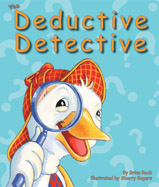Alignment to Standards for MN1 1.1.2.3 Recognize the relationship between counting and addition and subtraction. Skip count by 2s, 5s, and 10s.
1 1.2.2.1 Represent real-world situations involving addition and subtraction basic facts, using objects and number sentences.
1 1.2.2.4 Use addition or subtraction basic facts to represent a given problem situation using a number sentence.
2 2.1.2.1 Use strategies to generate addition and subtraction facts including making tens, fact families, doubles plus or minus one, counting on, counting back, and the commutative and associative properties. Use the relationship between addition and subtraction to
2 2.1.2.2 Demonstrate fluency with basic addition facts and related subtraction facts.
K K.1.2.1 find the sums and differences of numbers between 0 and 10.

Back to Standards Page